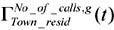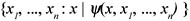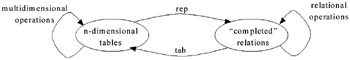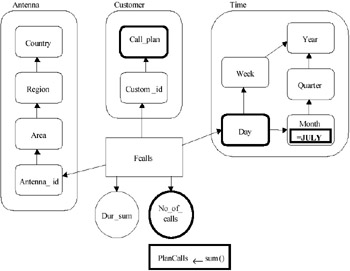# QUERY LANGUAGES BASED ON MULTIDIMENSIONAL MODELS

In this section we illustrate some of the most significant query languages for multidimensional data, based on specifically designed models, proposed in the literature. Since in most cases the models are based on an abstract data type, often called cube, we prefix the original term with a letter, in order to distinguish it from the general concept of cube used throughout this chapter and also to distinguish each "cube" from the "cubes" proposed by other authors. We therefore speak of G-cubes, S-cubes, etc. and also of f-dimensions, even if in the original literature they were simply referred to as cubes and dimensions. We do not consider here the standard operators of roll-up, drill-down, slice and dice, and pivot that have been covered in detail in an earlier chapter and which are obviously included in all models for multidimensional data. We focus instead on the peculiarities of each model and query language.

### A Grouping Algebra for Cubes

The G-cube schemes proposed in Li & Wang (1996) have several analogies with relational star schemes. As usual in data models, the authors distinguish n-dimensional G-cube schemes and G-cube instances (G-cubes for brevity). Each dimension is represented by a name and a collection of attributes (as in dimension tables of star schemes). G-cubes are defined by a collection of dimensions and by a function that maps combinations of dimension instances to values (measures) in a domain of scalar values (integers, reals, etc.).

The key features of the model are the distinction between conventional and dimension attributes, along with the concepts of grouping (relation) schemes and grouping relations, by means of which the authors introduce an operator which extends the conventional SQL GROUP BY clause. The operator combines the classic grouping capabilities of relational languages with those related to cube dimensions. The proposed query language is basically an extension of the relational algebra and comprises: i) order-oriented operations to express queries like "Give me the 10 most used antennas in 2001"; ii) aggregate operations exploiting the extended capabilities of the grouping operator.

For example a grouping relation g on the scheme (Customer_no, Town_resid) could be used to express the town of residence of each customer. Then the expressionrepresents for each town t the total number of calls made by customers resident in town t. The model assumes that each G-cube contains only one measure, thus we have supposed that a new cube No_of_calls has been defined from Fcalls by restricting only to that measure.

### An Algebra for "Symmetric" Cubes

As already stressed, a desirable property for multidimensional query languages is the symmetric treatment of dimensions and measures, particularly the capability to use measures to classify data, i.e., to transform one or more measures into a dimension. Generally speaking, there are some typical OLAP operations (e.g., roll-up and drill-down) for which a clear distinction between dimensions and measures makes the query expression simpler and allows association of straightforward semantics. On the other hand, there are classes of queries on multidimensional data that are more clearly expressible if dimensions and measures are treated as simple attributes, just like in the relational model.

An interesting approach to this issue is proposed in Agrawal, Gupta, & Sarawagi (1997): the data model is based on the S-cube, which is constituted by a triple of components:

1. A collection of dimensions.

2. A mapping from the Cartesian product of the dimension domains to an element domain (which can itself be multidimensional). The values in the element domain generally correspond to measures, and the domain can itself be either Boolean or n-dimensional.

3. A tuple of names describing the elements of the S-cube.

The query language is based on an algebra. The operators take S-cubes as operands and produce S-cubes as output. The peculiarity of the model is in the element domain, since dimensions can be pushed into it and measures can be pulled from it, thus providing a powerful mechanism to symmetrically deal with dimensions and measures. The proposed algebra is shown to be at least as powerful as the relational algebra, and several common OLAP operations (e.g., roll-up, drill-down, slice and dice, star join) can be easily expressed by the proposed operators.

### An MD Calculus for Fact Tables

The interaction between the user and a data warehouse is often based on a collection of operators that are applied on relations or cubes, and produce relations and cubes as output. In many cases, however, the use of a calculus for expressing queries is preferable, because it enables the user to express the manipulation in a more intuitive declarative way. The calculus MD-CAL proposed in Cabibbo & Torlone (1997) is based on a specific model for multidimensional data, called MD, which is closely related to snowflake schemes: fact tables and dimensions have a logical counterpart in the model, called f-tables and f-dimensions. Unlike most other multidimensional models, dimensions are described by normalized hierarchical structures, where the hierarchical relationships among dimension levels are modeled by roll-up functions R-UP.

MD-CAL queries define a mapping from instances over an input MD scheme to instances over an output MD scheme, where the input and the output schemes are defined over the same f-dimensions but distinct f-tables. Queries have the typical calculus form:where ψ is a first-order formula involving f-tables, roll-up functions, interpreted scalar functions (e.g., the standard arithmetic operators), and aggregate functions (e.g., min, max, sum, count, and avg). x is a special variable corresponding to the measure of the fact table and is called the result variable. The use of first-order expressions enables the symmetric treatment of measures and dimensions, i.e., the capability to transform measures into dimension components and vice versa, thus performing what the authors call an abstraction transformation.

### Querying by n-Dimensional Tables

An important drawback of several models for multidimensional data is that, although providing powerful constructs to express typical OLAP operations, they often require a complex syntax to express conceptually simple queries that are instead easily expressed by relational query languages. In Gyssens & Lakshmanan (1997), a model is proposed whose aim is to provide a conceptual description of multidimensional data, remaining as close to the standard relational model as possible, therefore minimizing the above-mentioned difficulties in expressing standard relational manipulations.

The model is based on a conceptual view of multidimensional data, which is closely related to star schemes. Particularly, an n-dimensional table schema is analogous to the (relational) schema of the dimension tables, and the instance of an n-dimensional table schema is analogous to the instances of both the dimension and the fact tables in a star schema. The key idea of the model is to define a one-to-one mapping between table schemes and relational schemes, based on a correspondence between tables and "completed" relations. This mapping enables the users to go back and forth from the tabular to the relational formalism, expressing the queries in the most suitable environment. Some classical OLAP operators are defined, particularly to express classifications and summarizations (aggregations), and it is shown that many others can be derived in terms of them, e.g., cube and monotone roll-up.

In order to express "relational" operations on (n-dimensional) tables, the tables are first transformed into relations (by using the function of relational representation rep) and then the conventional relational operators are applied. Conversely, multidimensional operations on relations can be easily expressed by first transforming the relations into tables (by the function of tabular representation tab) and then using the specific multidimensional operators.

The symmetric treatment of measures and dimensions is obtained by a technique similar to the one of the S-cubes. Two operators are defined that affect the structure of tables: by the unfold operator a set X of measure attributes can be "pulled" and used to constitute a new dimension, while the fold operator can be used to "push" dimensions into the measure components.

### Embedding Hierarchies in the Relational ModelFigure 3: Relational and Multidimensional Operations on Tables

All multidimensional models considered so far are based on the definition of an abstract data type corresponding to the concept of data cube. In Jagadish, Lakshmanan, & Srivastava (1999), a different approach is pursued: the relational model is considered and the introduced abstract data type refers to dimension hierarchies. This enables the authors to overcome some modeling limitations related to star and snowflake schemes, particularly to describe:

• Unbalanced hierarchies, i.e., such that some of the paths from the root to the leaf instances traverse all nodes of the hierarchy, while other paths only traverse a subset of them. This is a typical problem when considering territorial hierarchies in different countries because, for example, territories are classified in regions, states, counties, and cities in the U.S., but simply in regions and cities in a smaller country.

• Heterogeneous hierarchies, i.e., such that the leaf nodes (dimension levels) differ according to the instance of a dimension in an upper level, e.g., antennas may be classified by type, (digital or analog) but digital antennas are further classified according to the number of bits of the transmitted signal, while analog are further classified according to the bandwidth.

The model is called SQL(H) and the query language is basically an extension of SQL with two added features: i) the DIMENSIONS clause, which extends the FROM clause to dimension tables, and is used to specify dimension levels to be included in the multidimensional query; ii) a collection of hierarchical predicates to represent conditions like "A is a descendant of B in the dimension hierarchy."

The problem of heterogeneous hierarchies in OLAP systems has also been analyzed in Lehner (1998), where nested data cubes are proposed for modeling and querying.

### Visual Query Languages

Probably the first system enabling the user to query multidimensional (statistical) data by a graphical model was Subject (Chan & Shoshani, 1981). In Subject, multidimensional data are modeled by particular directed acyclic graphs (DAGs), which have some similarities with snowflake schemes. The nodes of the DAG have an inner label (X or C), representing the type of the node, and an external label representing its name. X nodes correspond to fact tables and some of the C nodes to dimension levels. Besides, C nodes are also used to group several X nodes just like "folders" of modern iconic operating systems. The query system is based on a hierarchical navigation on the DAGs, selecting the X node and the connected C nodes of interest (i.e., in the multidimensional terminology, the measures and the dimensions).

A similar approach is used in the ADAMO system, based on the homonymous graphical model for aggregate data (Rafanelli, Bezenchek, & Tininini, 1996): circle nodes and edges are used to represent dimension levels and hierarchies, while square nodes correspond to measures. Typical OLAP operations are directly performed on the graphical representations, e.g., adding a circle node to drill-down and removing it to roll-up or selecting specific values of a dimension level to slice and dice.

More recently, a visual query language was proposed (Cabibbo & Torlone, 1998a), based on some algebraic operators for the multidimensional model MD (Cabibbo & Torlone, 1998b). An elementary query for the running example used throughout this chapter is shown in Figure 4. It represents the query: "Give me the total number of calls in July, by day and call plan."Figure 4: A Graphical Query of the MD Model

The chosen measures and dimension levels are visually represented by thick lines. Selection conditions are directly represented on the corresponding level, and the aggregation to be performed for the roll-up is specified below the corresponding measure. Two or more queries of this form can be graphically composed (e.g., using scalar functions and aggregations) enabling the user to formulate arbitrarily complex queries.

### Multidimensional Queries on Statistical Databases

Similarities and differences between OLAP and statistical databases are the main focus of Chapter 2. Here, we only outline some peculiarities that need to be taken into account when designing an interface for statistical database querying.

One of the crucial issues for statistical databases is the representation of complex territorial hierarchies which evolve over time. The problems related to the temporal evolution of dimensions and dimension hierarchies are covered in detail in Chapter 6. Some solutions adopted in an implemented statistical information system can be found in Tininini et al. (2002).

Another important issue is related to the fact that in statistical databases the number of "points" of the multidimensional space, corresponding to data allowable for publication, is very small compared with the global size of the multidimensional space itself. In other words the multidimensional array representing allowable data in the global multidimensional space is sparse, and there are two main reasons for that:

• In statistical databases it is crucial to preserve the privacy of the individuals, and excessive detail in aggregate data can produce disclosure of sensitive information about single individuals (see Chapter 11).

• Much of the data in statistical databases originate from sample surveys, and the corresponding aggregate data are not significant for the finest levels of detail. Typically, a collection of data is considered to be significant only if the cardinality of each group individuated by the dimensional product (GROUP BY) is greater than a given threshold value.

As a consequence, an interface enabling the user to freely navigate on the dimension hierarchies would often lead the user to express a query, corresponding to non-allowable data. Specific techniques are consequently required to establish a trade-off between the characteristic freedom of multidimensional navigation and the constraints of significance and disclosure prevention imposed by statistical databases.Multidimensional Databases: Problems and Solutions
ISBN: 1591400538
EAN: 2147483647
Year: 2003
Pages: 150